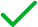# Indicators

## Plotting Indicators

### Introduction

LEAN provides helper methods to make it simple to create indicator plots.

### Plot Update Events

To plot all of the values of some indicators, in the Initialize method, call the PlotIndicator method. The method plots each indicator value as the indicator updates. The method accepts up to four indicators.

var symbol = AddEquity("SPY");
var smaShort = SMA(symbol, 10);
var smaLong = SMA(symbol, 20);
PlotIndicator("<chartName>", smaShort, smaLong)
symbol = self.AddEquity("SPY")
sma_short = self.SMA(symbol, 10)
sma_long = self.SMA(symbol, 20)
self.PlotIndicator("<chartName>", sma_short, sma_long)

### Plot Current Values

To plot the current value of indicators, call the Plot method. The method accepts up to four indicators.

// In Initialize
var smaShort = SMA(symbol, 10);
var smaLong = SMA(symbol, 20);

// In OnData
Plot("<chartName>", smaShort, smaLong)
# In Initialize
sma_short = self.SMA(symbol, 10)
sma_long = self.SMA(symbol, 20)

# In OnData
self.Plot("<chartName>", sma_short, sma_long)

### View Charts

The following table describes where you can access your charts, depending on how to deploy your algorithms:

LocationAlgorithm Lab AlgorithmsCLI Cloud AlgorithmsCLI Local Algorithms
Backtest results pageLive results page/backtests/read endpoint/live/read endpointReadBacktest methodReadLiveAlgorithm methodLocal JSON file in your <projectName> / backtests / <timestamp> or <projectName> / live / <timestamp> directory### Chart Limits

Not all indicators share the same base type(T), so you may not be able to plot them together since some indicators require points while others require TradeBars.

// Plot indicators that extend the "Indicator" type
PlotIndicators("All Indicator Values", sma, rsi);
Plot("Current Indicator Values", sma, rsi);

// Plot indicators that extend the "TradeBarIndicator" type
PlotIndicators("All Indicator Values", atr, aroon);
Plot("Current Indicator Values", atr, aroon);
# Plot indicators that extend the "Indicator" type
self.PlotIndicators("All Indicator Values", sma, rsi)
self.Plot("Current Indicator Values", sma, rsi);

# Plot indicators that extend the "TradeBarIndicator" type
self.PlotIndicators("All Indicator Values", atr, aroon)
self.Plot("Current Indicator Values", atr, aroon);


If your indicator plots are complex, call the Plot method with one indicator and plot its .Current.Value. For more information about plotting, see Charting.

You can also see our Videos. You can also get in touch with us via Discord.